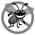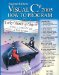# Formulating Algorithms: Nested Control Statements

### Formulating Algorithms Nested Control Statements

For the next example, we once again formulate an algorithm by using pseudocode and top-down, stepwise refinement, and write a corresponding C# application. We have seen that control statements can be stacked on top of one another (in sequence). In this case study, we examine the only other structured way control statements can be connected, namely, by nesting one control statement within another.

Consider the following problem statement:

A college offers a course that prepares students for the state licensing exam for real estate brokers. Last year, 10 of the students who completed this course took the exam. The college wants to know how well its students did on the exam. You have been asked to write an application to summarize the results. You have been given a list of these 10 students. Next to each name is written a 1 if the student passed the exam or a 2 if the student failed.

Your application should analyze the results of the exam as follows:

1. Input each test result (i.e., a 1 or a 2). Display the message "Enter result" on the screen each time the application requests another test result.
2. Count the number of test results of each type.
3. Display a summary of the test results indicating the number of students who passed and the number who failed.
4. If more than eight students passed the exam, print the message "Raise tuition."

After reading the problem statement, we make the following observations:

1. The application must process test results for 10 students. A counter-controlled loop can be used because the number of test results is known in advance.
2. Each test result has a numeric valueeither a 1 or a 2. Each time the application reads a test result, the application must determine whether the number is a 1 or a 2. We test for a 1 in our algorithm. If the number is not a 1, we assume that it is a 2. (Exercise 5.24 considers the consequences of this assumption.)
3. Two counters are used to keep track of the exam resultsone to count the number of students who passed the exam and one to count the number of students who failed the exam.
4. After the application has processed all the results, it must determine whether more than eight students passed the exam.

Let us proceed with top-down, stepwise refinement. We begin with a pseudocode representation of the top:

analyze exam results and decide whether tuition should be raised

Once again, the top is a complete representation of the application, but several refinements are likely to be needed before the pseudocode can evolve naturally into a C# application.

Our first refinement is

initialize variables
input the 10 exam results, and count passes and failures
print a summary of the exam results and decide whether tuition should be raised

Here too, even though we have a complete representation of the entire application, further refinement is necessary. We now specify individual variables. Counters are needed to record the passes and failures, a counter will be used to control the looping process and a variable is needed to store the user input. The variable in which the user input will be stored is not initialized at the start of the algorithm, because its value is read from the user during each repetition of the loop.

The pseudocode statement

initialize variables

can be refined as follows:

initialize passes to zero
initialize failures to zero
initialize student counter to one

Notice that only the counters are initialized at the start of the algorithm.

The pseudocode statement

input the 10 exam results, and count passes and failures

requires a loop that successively inputs the result of each exam. We know in advance that there are precisely 10 exam results, so counter-controlled looping is appropriate. Inside the loop (i.e., nested within the loop), a double-selection statement will determine whether each exam result is a pass or a failure and will increment the appropriate counter. The refinement of the preceding pseudocode statement is then

while student counter is less than or equal to 10
prompt the user to enter the next exam result
input the next exam result
if the student passed
else

We use blank lines to isolate the if...else control statement, which improves readability.

The pseudocode statement

print a summary of the exam results and decide whether tuition should be raised

can be refined as follows:

print the number of passes
print the number of failures

if more than eight students passed
print "Raise tuition"

Complete Second Refinement of Pseudocode and Conversion to Class Analysis

The complete second refinement of the pseudocode appears in Fig. 5.11. Notice that blank lines are also used to set off the while statement for readability. This pseudocode is now sufficiently refined for conversion to C#. The C# class that implements the pseudocode algorithm is shown in Fig. 5.12, and two sample executions appear in Fig. 5.13.

Figure 5.11. Pseudocode for the examination-results problem.

 ``` 1 initialize passes to zero 2 initialize failures to zero 3 initialize student counter to one 4 5 while student counter is less than or equal to 10 6 prompt the user to enter the next exam result 7 input the next exam result 8 9 if the student passed 10 add one to passes 11 else 12 add one to failures 13 14 add one to student counter 15 16 print the number of passes 17 print the number of failures 18 19 if more than eight students passed 20 print "Raise tuition" ```

Figure 5.12. Analysis of examination results, using nested control statements.

 ``` 1 // Fig. 5.12: Analysis.cs 2 // Analysis of examination results, using nested control statements. 3 using System; 4 5 public class Analysis 6 { 7 public void ProcessExamResults() 8 { 9 // initializing variables in declarations 10 int passes = 0; // number of passes 11 int failures = 0; // number of failures 12 int studentCounter = 1; // student counter 13 int result; // one exam result from user 14 15 // process 10 students using counter-controlled repetition 16 while ( studentCounter <= 10 ) 17 { 18 // prompt user for input and obtain value from user 19 Console.Write( "Enter result (1 = pass, 2 = fail): " ); 20 result = Convert.ToInt32( Console.ReadLine() ); 21 22 // if...else nested in while 23 if ( result == 1 ) // if result 1, 24 passes = passes + 1; 25 else // else result is not 1, so 26 failures = failures + 1; // increment failures 27 28 // increment studentCounter so loop eventually terminates 29 studentCounter = studentCounter + 1; 30 } // end while 31 32 // termination phase; prepare and display results 33 Console.WriteLine( "Passed: {0} Failed: {1}", passes, failures ); 34 35 // determine whether more than 8 students passed 36 if ( passes > 8 ) 37 Console.WriteLine( "Raise Tuition" ); 38 } // end method ProcessExamResults 39 } // end class Analysis ```

Figure 5.13. Test application for class Analysis.

(This item is displayed on pages 200 - 201 in the print version)

``` 1 // Fig. 5.13: AnalysisTest.cs
2 // Test application for class Analysis.
3 public class AnalysisTest
4 {
5 public static void Main( string[] args )
6 {
7 Analysis application = new Analysis(); // create Analysis object
8 application.ProcessExamResults(); // call method to process results
9 } // end Main
10 } // end class AnalysisTest
```

 ```Enter result (1 = pass, 2 = fail): 1 Enter result (1 = pass, 2 = fail): 2 Enter result (1 = pass, 2 = fail): 1 Enter result (1 = pass, 2 = fail): 1 Enter result (1 = pass, 2 = fail): 1 Enter result (1 = pass, 2 = fail): 1 Enter result (1 = pass, 2 = fail): 1 Enter result (1 = pass, 2 = fail): 1 Enter result (1 = pass, 2 = fail): 1 Enter result (1 = pass, 2 = fail): 1 Passed: 9 Failed: 1 Raise Tuition ``` ```Enter result (1 = pass, 2 = fail): 1 Enter result (1 = pass, 2 = fail): 2 Enter result (1 = pass, 2 = fail): 2 Enter result (1 = pass, 2 = fail): 2 Enter result (1 = pass, 2 = fail): 1 Enter result (1 = pass, 2 = fail): 1 Enter result (1 = pass, 2 = fail): 1 Enter result (1 = pass, 2 = fail): 1 Enter result (1 = pass, 2 = fail): 2 Enter result (1 = pass, 2 = fail): 2 Passed: 5 Failed: 5 ```

Lines 1013 of Fig. 5.12 declare the variables that method ProcessExamResults of class Analysis uses to process the examination results. Several of these declarations use C#'s ability to incorporate variable initialization into declarations (passes is assigned 0, failures is assigned 0 and studentCounter is assigned 1). Looping applications may require initialization at the beginning of each repetitionsuch reinitialization would normally be performed by assignment statements rather than in declarations.

The while statement (lines 1630) loops 10 times. During each repetition, the loop inputs and processes one exam result. Notice that the if...else statement (lines 2326) for processing each result is nested in the while statement. If the result is 1, the if...else statement increments passes; otherwise, it assumes the result is 2 and increments failures. Line 29 increments studentCounter before the loop condition is tested again at line 16.

After 10 values have been input, the loop terminates and line 33 displays the number of passes and the number of failures. The if statement at lines 3637 determines whether more than eight students passed the exam and, if so, outputs the message "Raise Tuition".## Error Prevention Tip 5 4

Initializing local variables when they are declared helps you avoid compilation errors that might arise from attempts to use uninitialized data. While C# does not require that local variable initializations be incorporated into declarations, it does require that local variables be initialized before their values are used in an expression.

AnalysisTest Class That Demonstrates Class Analysis

Class AnalysisTest (Fig. 5.13) creates an Analysis object (line 7) and invokes the object's ProcessExamResults method (line 8) to process a set of exam results entered by the user. Figure 5.13 shows the input and output from two sample executions of the application. During the first sample execution, the condition at line 36 of method ProcessExamResults in Fig. 5.12 is truemore than eight students passed the exam, so the application outputs a message indicating that the tuition should be raised.

### Compound Assignment OperatorsVisual C# 2005 How to Program (2nd Edition)
ISBN: 0131525239
EAN: 2147483647
Year: 2004
Pages: 600